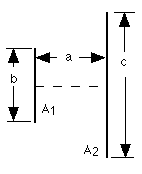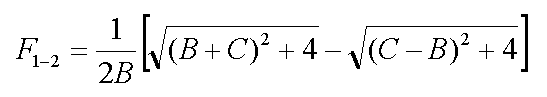Cody

# Problem 2806. Radiation Heat Transfer — View Factors (2)

View factors (aka configuration factors) are utilized in some radiation heat transfer models to estimate heat transfer rates between surfaces. In particular, the thermal energy leaving a given surface is applied to other surfaces, as appropriate, based on how much the hot surface "sees" the other surfaces. As such, view factors are purely geometrical in nature. A range of view factor formulae are available here.

For this problem, calculate the view factor from surface 1 to surface 2 (F_1-2) for two directly opposed, infinitely long plates of different finite widths given the distance between the plates:Each variable may be a vector of values. Also, note that B = b/a and C = c/a.

### Solution Stats

36.0% Correct | 64.0% Incorrect
Last Solution submitted on Oct 04, 2019